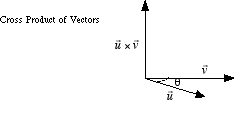# cross product

Cross Product

A way of multiplying two vectors, written u × v, in which the product is another vector. The cross product of two vectors results in a vector which is orthogonal to both the vectors being multiplied. The magnitude of the cross product of two vectors is found by the formula|u × v| = |u||v| sin θ, where θ is the smaller angle between the vectors.

Note: Cross products are not commutative. That is, u × vv × u. The vectors u × v and v × u have the same magnitude but point in opposite directions.